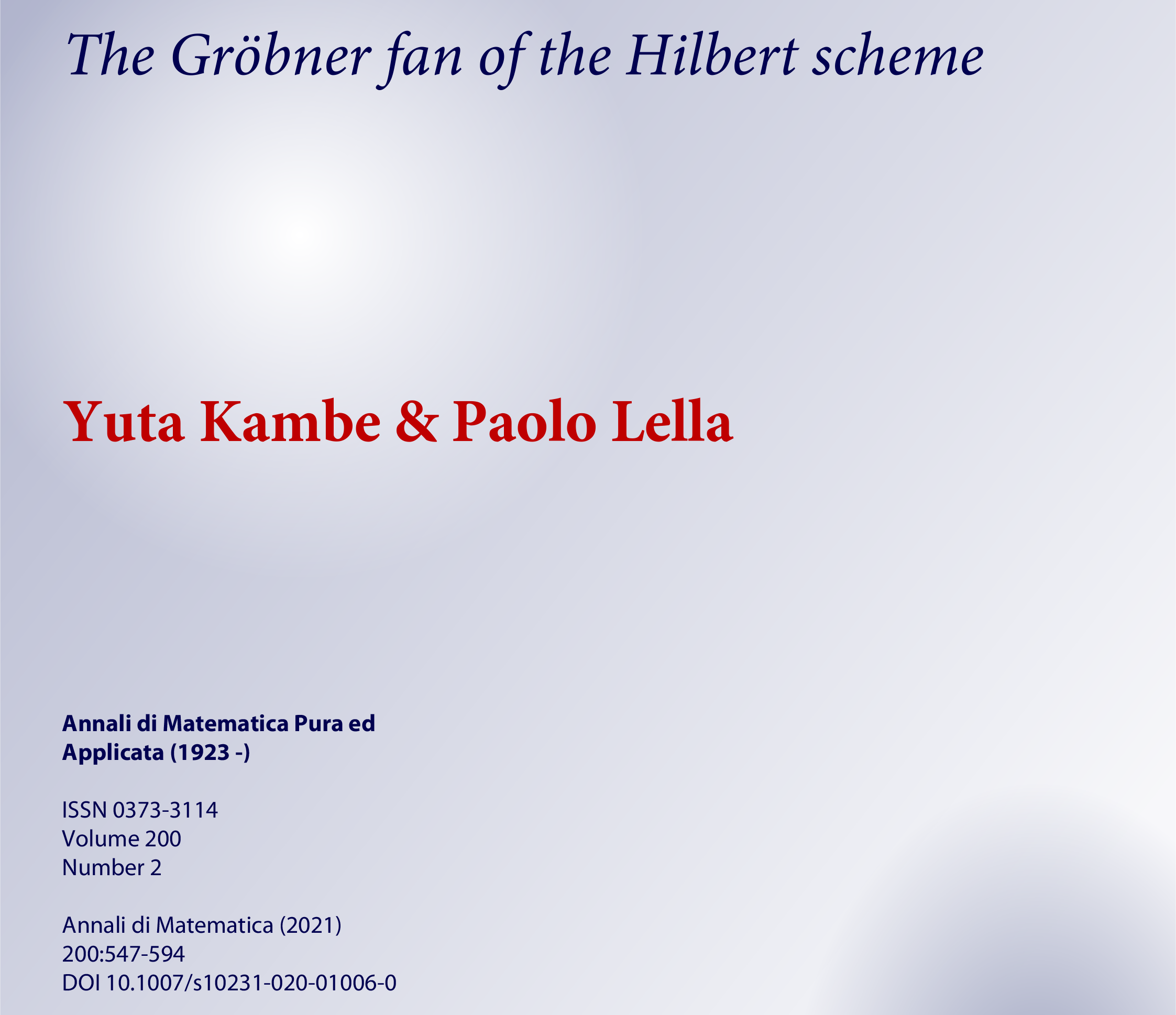### The Gröbner fan of the Hilbert scheme

##### Annali di Matematica Pura ed Applicata, 200:547–594, 2021.

Parole chiave

Abstract. We give a notion of "combinatorial proximity" among strongly stable ideals in a given polynomial ring with a fixed Hilbert polynomial. We show that this notion guarantees "geometric proximity" of the corresponding points in the Hilbert scheme. We define a graph whose vertices correspond to strongly stable ideals and whose edges correspond to pairs of adjacent ideals. Every term order induces an orientation of the edges of the graph. This directed graph describes the behavior of the points of the Hilbert scheme under Gröbner degenerations with respect to the given term order.

Then, we introduce a polyhedral fan that we call Gröbner fan of the Hilbert scheme. Each cone of maximal dimension corresponds to a different directed graph induced by a term order. This fan encodes several properties of the Hilbert scheme. We use these tools to present a new proof of the connectedness of the Hilbert scheme. Finally, we improve the technique introduced in the paper "Double-generic initial ideal and Hilbert scheme" by Bertone, Cioffi and Roggero to give a lower bound on the number of irreducible components of the Hilbert scheme.

© 2021 GAA@polimi Generato con Hugo e inspirato al tema Resume Ultimo aggiornamento: 8 Giugno 2022